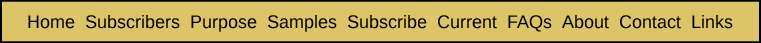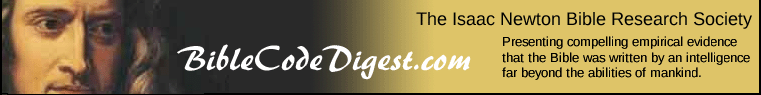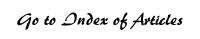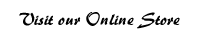Estimating the Expected Number of Occurrences of a Given ELS within a Specific Text Appendix Two from Bible Code Bombshell Now that we have derived a formula for the total number of ELSs with L skips and various intervals within a text of T letters, we are in a position to derive the expected number of occurrences of a specific sequence of letters as an ELS. That number will be the product of the probability that a given ELS will consist of the given sequence of (L+1) letters, times the total number of ELSs that can be formed with L skips. First, we will derive a formula for the probability part of the equation. Suppose that our text is the first 50 letters of Lincoln's Gettysburg Address. With spaces eliminated, it would look like this: fourscoreandsevenyearsagoourfathersbroughtforthont Then suppose we compile a table showing the total number of times that each letter of the alphabet appears. We can then divide the total number of appearances of each letter by the total number of letters (50) in this opening section of Lincoln's speech to obtain estimates of the probability of any letter turning out to be the given letter. We would then have Table App2A: Table App2A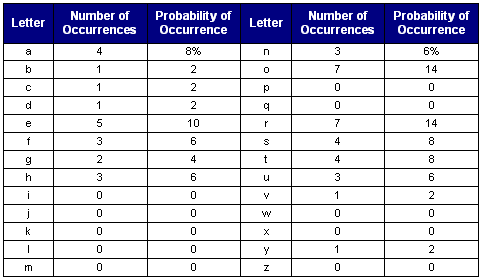Now suppose that we want to find out what the chances are that a randomly selected ELS of 10 letters (with some interval i and starting letter) taken from the above excerpt from Lincoln's speech were to spell out, "gettysburg." That would be estimated by taking the product of the probabilities in the above table. Suppose that we denoted the probability of a given letter being an m as P(m), then the chances we are estimating are: P(g) * P(e) * P(t) * P(t) * P(y) * P(s) * P(b) * P(u) * P(r) * P(g), or 4% * 10% * 8% * 8% * 2% * 8% * 2% * 6% * 14% * 4%% = 0.000000000000275%. This percentage is the same as the odds being 1 out of 3,633,045,014,881 or 1 out of 3.6 trillion. This is such an incredibly small probability that we might jump to the conclusion that it would be virtually impossible to find any ELS that spelled "gettysburg." However, to determine the expected number of times that "gettysburg" might occur as an ELS, we need to multiply the above percentage odds by the total number of possible ELSs that can be formed using 10 letters out of a text of length 50, with any interval possible and with both forward and backward ELSs permitted. We can calculate that number by using Formula 1C. We get 230 possible ELSs, including both forward and backward sequences. This results in an expected number of occurrences of the ELS of "gettysburg" within the first 50 letters of Lincoln's address of 0.0000000000633. This is about the same as the odds being 1 out of 15.8 billion that it would occur by chance. Obviously, this is extremely unlikely. If it were to occur, we would strongly suspect that Lincoln had deliberately constructed his speech to contain the ELS of "gettysburg" within it. If it is done deliberately, making one such insertion would not be that hard to do. It would be much like constructing part of a crossword puzzle. What would the likelihood be of finding "gettysburg" as an ELS within a text the length of a typical book? Suppose that book contained 500,000 letters, which would be about average for a book of 250 pages in length. Again, if we take the product of the probability of any given ELS spelling "gettysburg" times the total possible number of ELSs with 10 letters (or nine skips) within the entire text of 500,000 letters, we obtain an expected number of occurrences of the ELS of "gettysburg" within the given text. Using Formula 1C from the previous appendix, we conclude that there are 27,777,277,780, or 27.8 billion possible ELSs of nine skips that could be formed. When we multiply this number times the probability that any given ELS will spell "gettysburg," we come up with an expected number of occurrences within the 250 pages of text of 0.00765: (1 out of 3.6 trillion) * (27.8 billion) = 0.00765. This is about the same as saying that the odds of it occurring by chance are 1 out of 131 (=1/.00765), or somewhat less than 1 in 100, or 1%. If we were to expand our search to include 12 books of 500,000 letters in length, we would have 3,999,994,000,002 (or 4 trillion) possible ELSs. When we multiply this number by the probability of any given ELS spelling "gettysburg," we come up with 1.1 expected occurrences. In other words, the chances are quite high that it would crop up by coincidence within the text of 12 books of typical length. Now we all know that within the given 12 books, the ELS of "gettysburg" will either not appear at all, or will appear perhaps once, or twice, or maybe even three times or more. So, how can we interpret this finding that there are 1.1 expected occurrences? Mathematicians use a probability distribution called the Poisson distribution to determine the probability of any given number (k) of occurrences of an event, given that its expected number of occurrences (E) is known. That probability is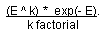In the above expression, k factorial is the product of the number k itself times every whole number smaller than k. So 3 factorial is equal to 3 times 2 times 1, or 6. And 5 factorial is 5 times 4 times 3 times 2 times 1, or 120. What is exp(-E)? Exp (E) is defined as Euler's constant (e), or 2.7182849, raised to the E-th power. Using the Poisson distribution, we can estimate the probability of any number of actual occurrences of the ELS of "gettysburg" within 12 books of average length, given that the ELS has an expected number of occurrences of 1.1 (E). This is given to us by Table App2B. Table App2B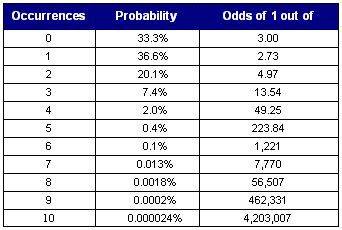Now suppose we consider the ELS of "abe." The probability of any given ELS spelling "abe" is P(a) * P(b) * P(e) = 8% * 2% * 10% = 0.00016%, or 1 out of 6,250. There are 4,900 possible ELSs that can be formed using three letters within a text of 50 letters. Taking the product of the probability of any ELS being "abe" times the total number of possible ELSs gives us 0.784 expected occurrences. Using the Poisson distribution this gives us the following probabilities for the number of occurrences of the ELS of "abe" (Table App2C): Table App2C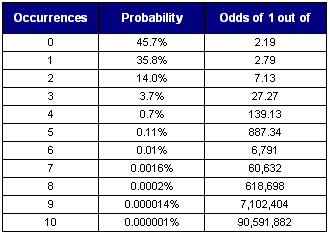So this tells us that the odds are almost fifty-fifty that the ELS of "abe" won't appear at all, about 1 out of 3 that it will occur once and about 1 out of 7 that it will appear twice. If the ELS "abe" were to appear 10 times within the first 50 letters of the Gettysburg Address, we would suspect Lincoln had embedded his name as a code within his speeches. After all, the odds of that happening by chance are almost 1 in 100 million! Some have argued that it is possible to find whatever you want as an ELS in the Torah. This question can be answered definitively on the basis of the types of formulas discussed in this appendix. Earlier we described a formula that mathematicians commonly use for determining the probability of a given number of occurrences of something � given that we know what the expected number of occurrences is. That formula is given by the Poisson distribution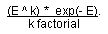What then is the probability that k will be zero, given E expected occurrences? By substituting k=0 in the above formula, we get exp(-E), since E^0 = 1 and [0 factorial] also equals 1. So if we can somehow determine E, the expected number of occurrences of an ELS, we can then calculate exp(-E) to obtain the chances that the ELS will simply not occur at all within a given text. The expected number of occurrences of an ELS in a given text can be derived as the product of the total possible number of ELSs times the probability that any single given ELS will have the exact sequence of letters that we have selected. For example, suppose there are 1 billion possible ELSs and that the chances that any given ELS is the one we are looking for are 1 in 100 million. Then we would expect that ELS to occur 10 times in the text since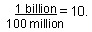How can we determine what is the total possible number of ELSs? Formula 1F from Appendix One provides the answer. How can we determine the chances that any single given ELS will have the exact sequence of letters we have chosen? That has been the subject of this appendix. Now suppose that we take the simplifying assumption that all 22 Hebrew letters are equally likely to occur. This, of course, is not true, but we are assuming it for the sake of discussion. Then the number of letter combinations that can be formed when there are A letters is simply 22^A. In other words, if there are 5 letters, there are 22^5 possible letter combinations, or 22 x 22 x 22 x 22 x 22 possible different strings of letters. Using this formula and the table from Appendix One for the total possible number of ELSs, we arrive at the following expected number of occurrences of any ELS, given how many letters it has (Table App2D). Table App2D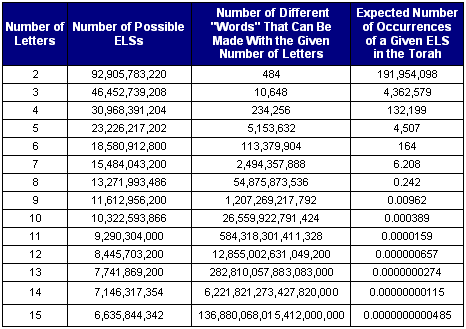If we now use the expected number of occurrences in the last column of Table App2D and apply the formula of exp(-E), we get Table App2E. Table App2EThese are highly conclusive � and intriguing � results. In summary, if an ELS has six or fewer letters, then it is basically certain that that ELS will appear at least once in the Torah. In other words, for such short ELSs it is true that you can find whatever you are looking for. If the ELS has seven letters, then there is a 1 in 500 chance that the ELS will simply not appear anywhere in the Torah. If the ELS has eight letters, there is nearly an 80% chance that it will not appear anywhere in the Torah. If it has nine letters, chances are 99% that it won't appear anywhere. And the more letters the ELS has above nine, it becomes increasingly unlikely that it can be found anywhere in the Torah. In conclusion, the assertion that you can find anything you want as an ELS in the Torah is true if the ELS has six or fewer letters, and is basically false if the ELS has eight or more letters. If we can find any intelligible ELSs with 10 or more letters, then we have discovered something that is quite unlikely to occur by chance. As any reader of Michael Drosnin's book may note, many of his codes have only three or four or five letters and so are basically certain to appear at least somewhere in the Torah. However, what is also typically a part of most of the examples in his book is that appearances of an ELS must also somehow be "near" other related ELSs or sections of text. In the next four appendices, we will be covering ways to estimate the probability that such occurrences could happen by chance.

 Enjoy finding your own Bible codes. Bible code search software is available in our online store. Subscribe Free! By signing up to be a member of The Isaac Newton Bible Research Society, you will have access to more than fifteen years of research by our team of Bible code researchers. Sign up to be a member today.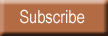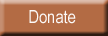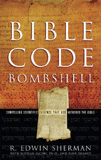Bombshell examines two massive, recently discovered clusters of codes in the Hebrew Old Testament. To read more about Bombshell, click here, or click below to order from Amazon today!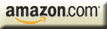| HOME | SUBSCRIBERS ONLY | OUR PURPOSE | FREE SAMPLES | CURRENT | | FAQs | ABOUT BIBLE CODES | SUBSCRIBE | CONTACT | Copyright © 2016 BibleCodeDigest.com